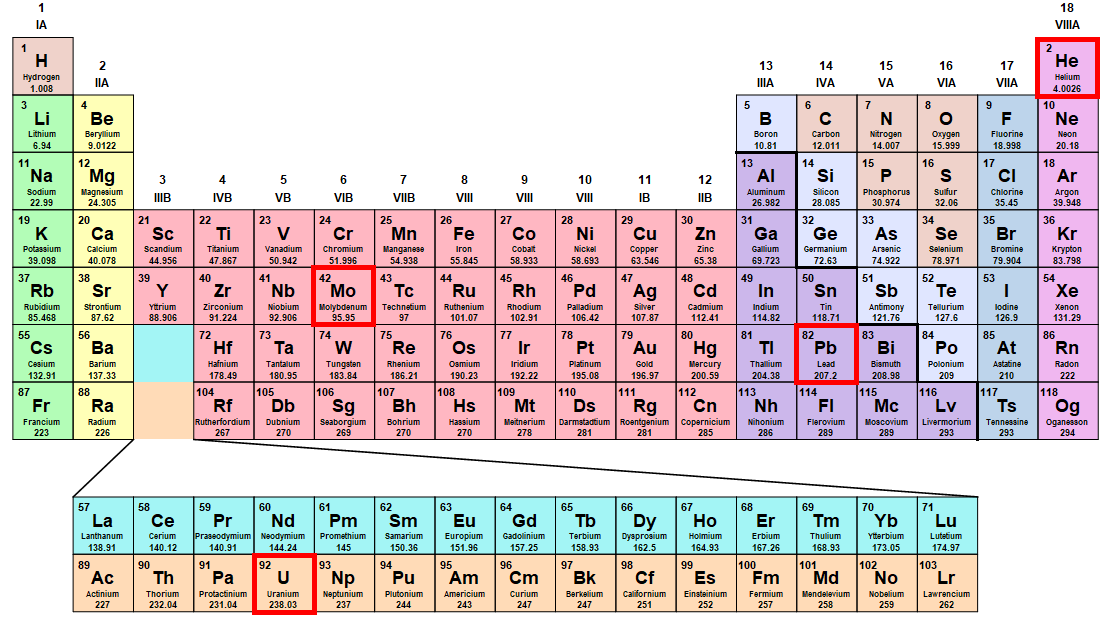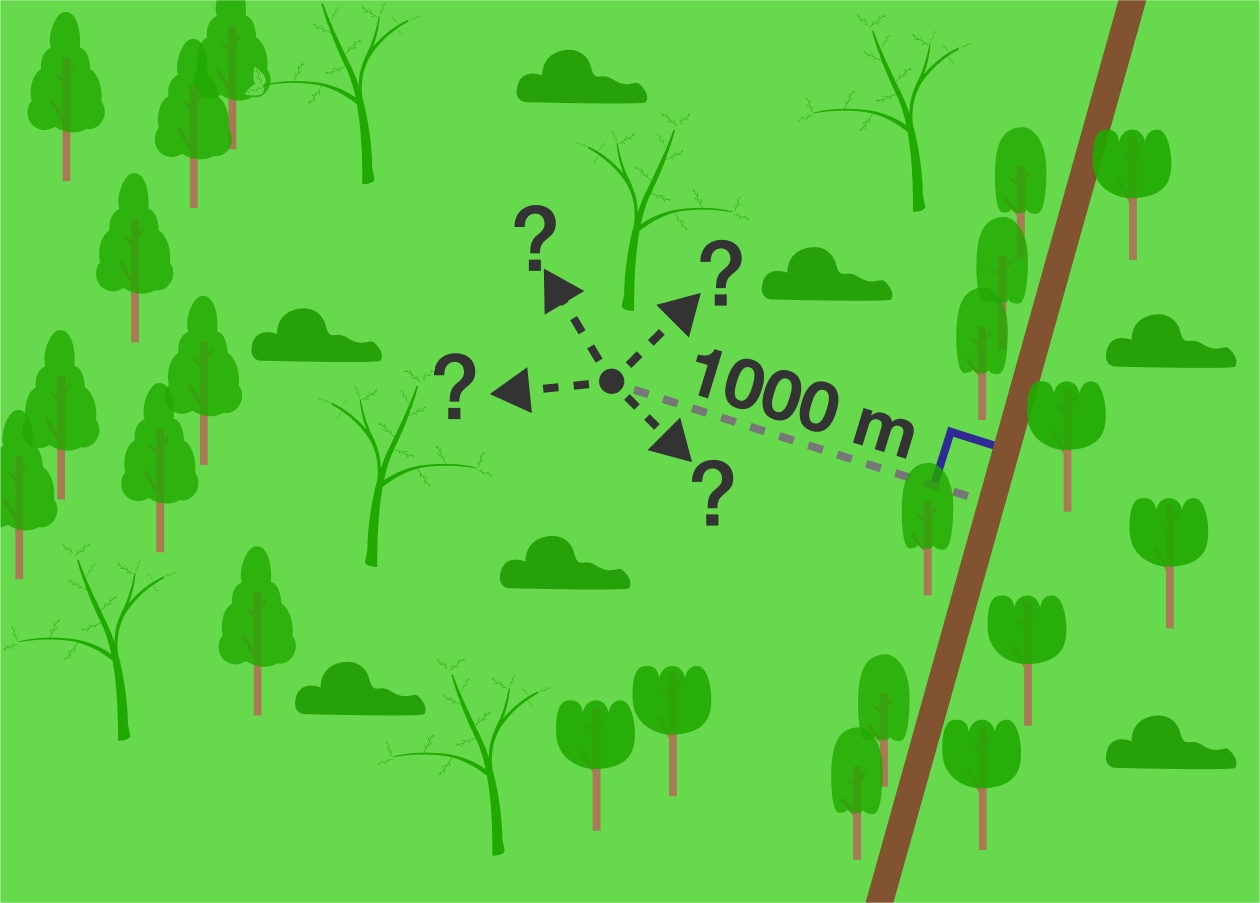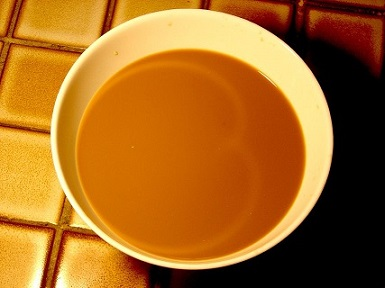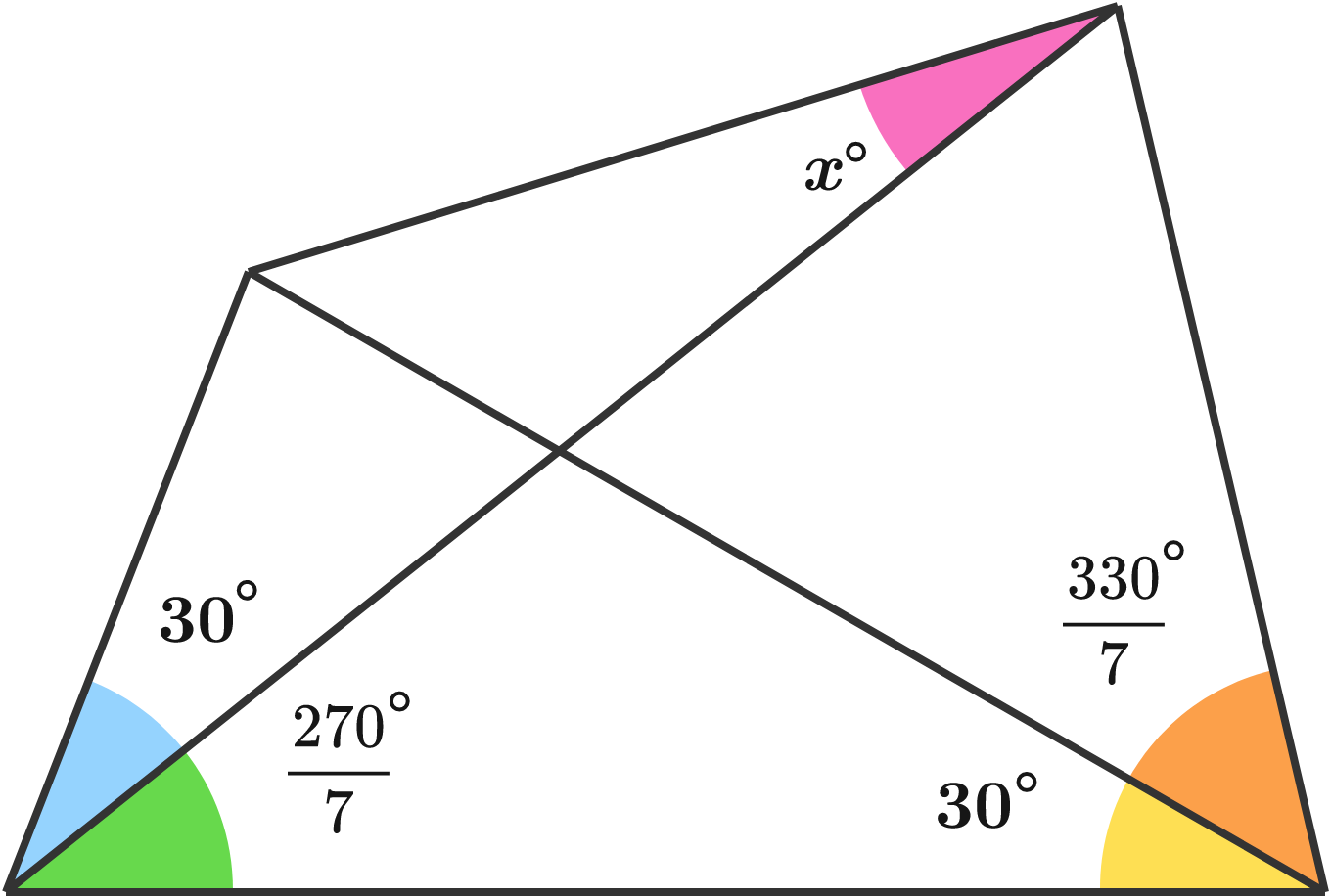# Problems of the Week

Contribute a problem

# 2018-06-11 Advanced

The founders of quantum mechanics largely ignored relativity when they first applied quantum theory to chemistry:

"The general theory of quantum mechanics is now almost complete... they give rise to difficulties only when high-speed particles are involved, and are therefore of no importance in the consideration of atomic and molecular structure and ordinary chemical reactions." - Paul Dirac, 1929

Treat an atom as a two-body problem with one electron and a central nucleus with a charge $$Z$$ and a mass much greater than the electron.

Was Dirac correct? At what element in the periodic table would relativistic effects become important for an electron in a ground state?Note: Significant errors start to appear between classical and relativistic dynamics when the Lorentz factor $$\gamma = \frac{1}{\sqrt{1-v^2/c^2}} \geq 1.05$$. It may be useful to perform this calculation in atomic units, where $$\hbar, e, m_e,$$ and $$4 \pi \epsilon_0$$ are defined as $$1.$$ In these units, the speed of light is $$c = 137$$.

You’re lost in the woods, and your only hope of reaching civilization is to find your way back to the only road.

• You know you are exactly 1000 meters from the infinitely long, straight road.
• You have perfect relative bearings, are able to track your position perfectly, and can travel along any line or curve.
• You don't know the direction of the road and cannot see it until you are upon it.

What is the shortest possible walking distance, to the nearest meter, that will ensure you reach the road?Be warned, your initial thoughts may need to be refined to truly minimize the length of your path.

Within a $$100 \times 100$$ square, draw in a shape that maximizes the ratio $$\frac{ \text{ Area } } { \text{ Perimeter } }$$.

What is this ratio (to 2 decimal places)?

For example, if we drew in a $$100 \times 100$$ square, we would get $$\frac{ 100 ^ 2 } { 4 \times 100 } = 25$$.The caustic appearing on the circular surface with radius $$3\text{ cm}$$ of this cup of coffee, with a spotlight source on the perimeter, turns out to be a cardioid.

What is the area $$\big($$in $$\text{cm}^2\big)$$ of the region that the cardioid encloses?

$$x$$ can be expressed as $$\frac{a}{b}$$, where $$a$$ and $$b$$ are coprime positive integers.

What is $$a+b?$$Note: Synthetic proofs welcome.

×

Problem Loading...

Note Loading...

Set Loading...